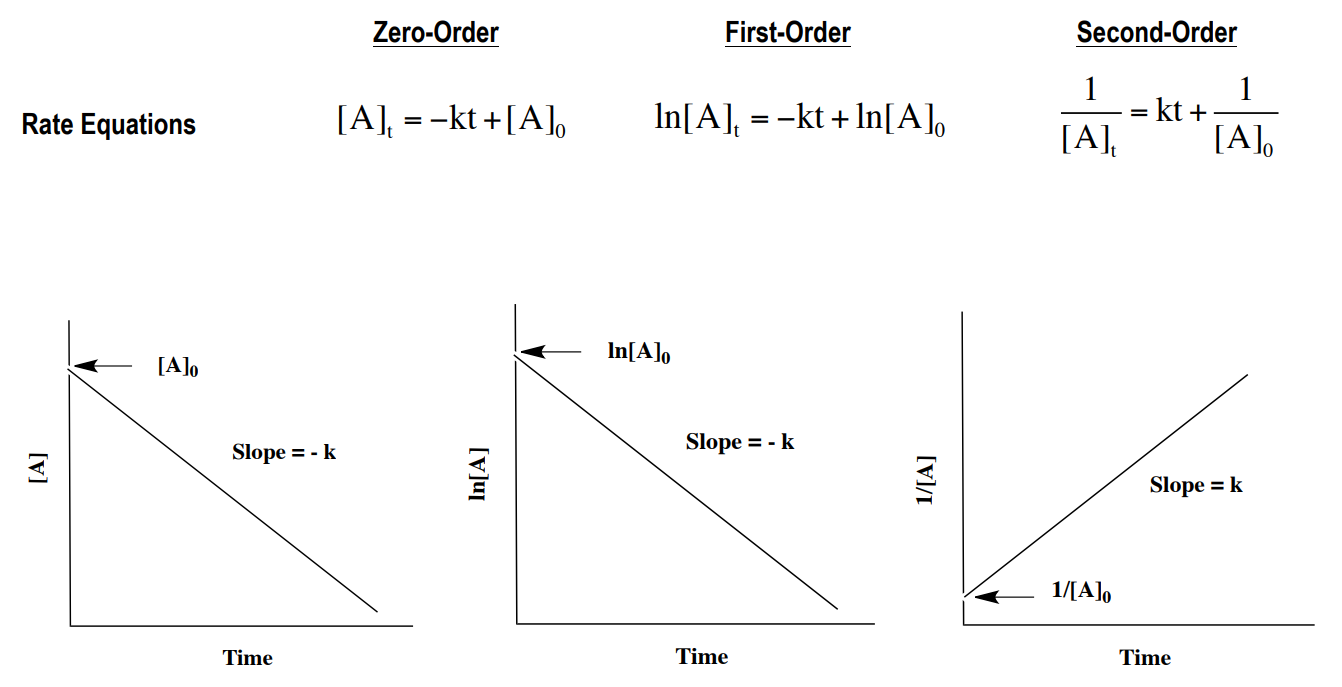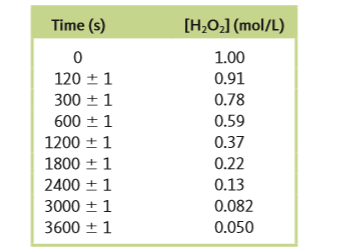# Problem: The decomposition of hydrogen peroxide was studied, and the following data were obtained at a particular temperature:Assuming thatRate = - Δ [H2O2] / Δtdetermine the rate law, the integrated rate law, and the value of the rate constant. Calculate [H2O2] at 4000. s after the start of the reaction.

###### FREE Expert SolutionWe need to plot the points according to the equations above and identify which is linear.

• Since plotting on a graph can be a bit unreliable when using only pen and paper we will use the stat function of the calculator.
• We can't really see the graph but we have to calculate for R2
• An R2 that is very close to 1 (about 0.99) should mean that the plot is linear.

99% (57 ratings)###### Problem Details

The decomposition of hydrogen peroxide was studied, and the following data were obtained at a particular temperature:Assuming that

Rate = - Δ [H2O2] / Δt

determine the rate law, the integrated rate law, and the value of the rate constant. Calculate [H2O2] at 4000. s after the start of the reaction.

Frequently Asked Questions

What scientific concept do you need to know in order to solve this problem?

Our tutors have indicated that to solve this problem you will need to apply the Identifying Reaction Order concept. If you need more Identifying Reaction Order practice, you can also practice Identifying Reaction Order practice problems.

What professor is this problem relevant for?

Based on our data, we think this problem is relevant for Professor Berg's class at UNLV.

What textbook is this problem found in?

Our data indicates that this problem or a close variation was asked in Chemistry: An Atoms First Approach - Zumdahl Atoms 1st 2nd Edition. You can also practice Chemistry: An Atoms First Approach - Zumdahl Atoms 1st 2nd Edition practice problems.